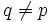# Hall subgroups exist in finite solvable group

Jump to: navigation, search

## Statement

Suppose$G$ is a finite solvable group, and$\pi$ is a prime set. Then, there exists a$\pi$-Hall subgroup (?) of$G$: a subgroup whose order and index are relatively prime, and with the property that the set of prime divisors of its order is within$\pi$.

## Proof

Given: A finite solvable group$G$, a prime set$\pi$.

To prove:$G$ has a$\pi$-Hall subgroup.

Proof: We prove the claim by induction on the order of$G$. The base case of the trivial group is clear.

Suppose the result is true for all finite solvable groups of order strictly less than the order of$G$. Then, by facts (1) and (2), the result is true for all proper subgroups and all proper quotients of$G$.

Let$N$ be a minimal normal subgroup of$G$. By fact (3),$N$ is elementary Abelian, and in particular, is a$p$-group for some prime divisor of$G$. Observe that:

1. Case$p \in \pi$: By applying induction to$G/N$, we conclude that$G/N$ has a$\pi$-Hall subgroup. Taking the inverse image of this in$G$, we get a$\pi$-Hall subgroup of$G$.
2. Case$p \notin \pi$: By applying induction to$G/N$, we conclude that$G/N$ has a$\pi$-Hall subgroup. The inverse image of this gives a subgroup, say$K$, of$G$. If$G/N$ is not itself a$\pi$-group, then$K/N$ is proper in$G/N$, and$K$ is a proper subgroup of$G$, then$K$ has a$\pi$-Hall subgroup$H$. Note that$[G:K] = [G/N:K/N]$ is relatively prime to$\pi$, and$[K:H]$ is relatively prime to$\pi$, so$[G:H]$ is relatively prime to$\pi$. Thus,$H$ is a Hall$\pi$-subgroup of$G$.

Thus, the only case of concern is where$p \notin \pi$ but$G/N$ is a$\pi$-group. Note that in this case,$N$ must be a$p$-Sylow subgroup (since$G/N$ has order relatively prime to$p$).

Let$M$ be a subgroup of$G$ such that$M/N$ is a minimal normal subgroup of$G/N$. Then, since the order of$G/N$ is not a multiple of$p$, and$G/N$ is solvable, fact (3) yields that$M/N$ is an elementary Abelian$q$-group for some prime$q \ne p$. By fact (5),$M$ is itself a normal subgroup of$G$.

Let$Q$ be a$q$-Sylow subgroup of$M$ (using fact (4)).

Now, if$Q$ is normal in$G$, then argue as before replacing$N$ by$Q$. Note that by our assumption,$q \in \pi$, so we land up in case (1).

Let's now consider the case that$Q$ is not normal in$G$. By Frattini's argument (fact (6)),$MN_G(Q) = G$. The product formula yields:$\frac{|G|}{|N_G(Q)|} = \frac{|M|}{|M \cap N_G(Q)|}$$Q \le M \cap N_G(Q)$, so$|M|/(|M \cap N_G(Q)|)$ divides$|M|/|Q|$, and is hence a power of$p$. Thus,$|G|/|N_G(Q)|$ is a power of$p$. Thus,$N_G(Q)$ is a proper subgroup of$G$ whose index is a power of$p$. By induction,$N_G(Q)$ has a$\pi$-Hall subgroup, and this must also be a$\pi$-Hall subgroup for$G$.